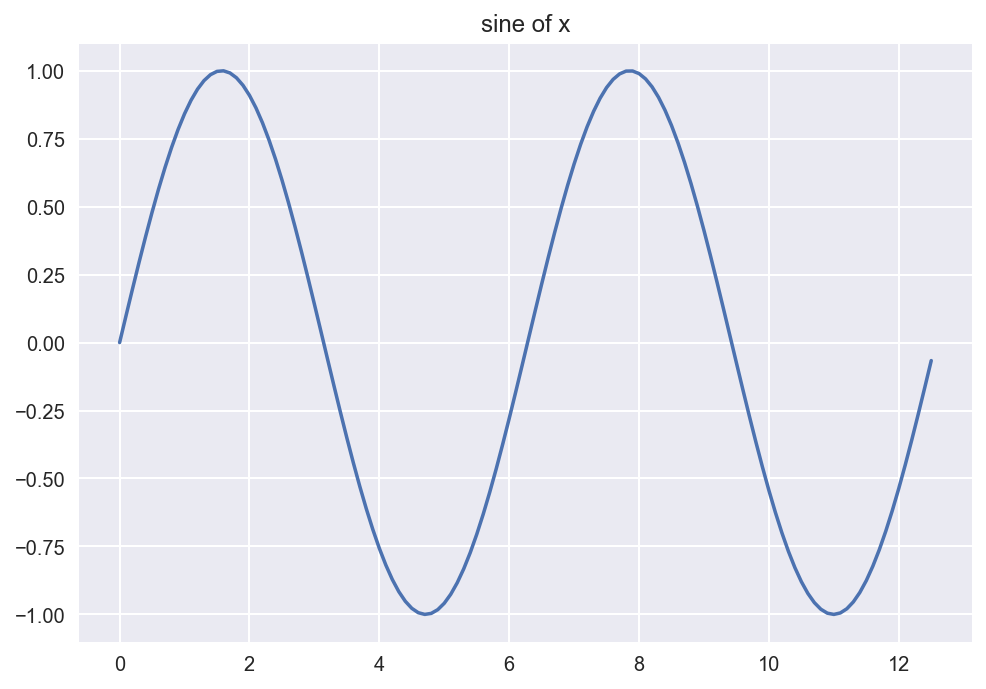nb_markdown

# NOTEBOOK MARKDOWN TUTORIAL¶

How to insert an image, resize, and align (suppose the name of the image file is "Banner_MATH2319.png" in the same directory as in this Jupyter notebook):

<img src="./Banner_MATH2319.png" width=400 align="left"/>## Prep for Plotting ¶

In :
import numpy as np
import matplotlib.pyplot as plt

%matplotlib inline
%config InlineBackend.figure_format = 'retina'
plt.style.use('seaborn')


## How to Plot the Sine Function ¶

In :
x = np.arange(0, 4*np.pi, 0.1)

In :
y = np.sin(x)

In :
plt.plot(x,y)
plt.title('sine of x')
plt.show()## Working with Markdown Commands ¶

This is a block quote.

This is a line break:

This is code section example:

a = 23
b = 15
print(a+b)


Here is a line break
that looks nice.

Emphasis: Everybody likes machine learning.

This is italic.

This is bold.

Here is an unordered list:

• Item 1
• Item 2
• Item 3

Here is an ordered list:

1. Item 1
2. Item 2
3. Item 3

Here is an ordered list with sublists:

1. Item 1
• Item 1A
• Item 1B
• Item 1B.1
• Item 1B.2
• Item 1C
• Item 1D
2. Item 2
3. Item 3

How to create a table:

Course Name Code Level
Machine Learning 2319 PG
Intro to Analytics 2350 UG

This is a famous formula: $E=mc^2$

Here is a centered math formula: $$\sum_i^{j+k} x^3= \frac{\alpha}{\beta}$$

External webpage links: Our website is here.

www.featureranking.com